• =SUMPRODUCT(($C$1:$C$10000="hete->s288c")*($H$1:$H$10000s288c")*($H$1:$H$10000 =SUMPRODUCT(($C$1:$C$100000045000)...条件的求和 复制excel中的公式，而不是公式后得到的结果 假设公式在 A列 选中A列, 按

=SUMPRODUCT(($C$1:$C$10000="hete->s288c")*($H$1:$H$10000<900))-SUMPRODUCT(($C$1:$C$10000="hete->s288c")*($H$1:$H$10000<895))

=SUMPRODUCT(($C$1:$C$1000000<50000)*($C$1:$C$1000000>45000)*$U$1:$U$1000000)
条件的求和

复制excel中的公式，而不是公式后得到的结果
假设公式在 A列
选中A列,  按Ctrl+~     数据---分列----固定宽度---下一步------下一步      列数据格式  选择  文本-----完成  ------ 按Ctrl+~

25
大于30且小于75的单元格个数
30
44
结果
公式
11

8

=SUM(IF(($V$4:$V$75<30)*($V$4:$V$75>0),1,0))
13
8
=COUNTIF(V4:V75,">=0")-COUNTIF(V4:V75,">=30")
-3
8
=SUMPRODUCT((V4:V75<30)*(V4:V75>0))
6
8
=SUM((V4:V75>0)*(V4:V75<30))
45
-7
20
26
35
数组在输入完成后要同时按Ctrl+Shift+Enter进行确定.
20
30
99
-7
19

SUMPRODUCT函数可以方便地计算工作表内多列中对应值相乘后的和，其语法为：
SUMPRODUCT(array1,array2,array3, …)其中，Array1, array2, array3, …为 2到 30个数组，其相应元素需要进行相乘并求和。数组参数必须具有相同的维数，否则，函数
SUMPRODUCT将返回错误值 #VALUE!。该函数将非数值型的数组元素作为 0处理。下面通过示例介绍SUMPRODUCT函数的基本使用方法。如下图1所示的工作表：

图1其中所定义的名称为：

名称

引用范围

公司

=Sheet1!$D$3:$D$17

全部数据

=Sheet1!$A$2:$E$17

日期

=Sheet1!$A$3:$A$17

姓名

=Sheet1!$B$3:$B$17

性别

=Sheet1!$C$3:$C$17

用工数

=Sheet1!$E$3:$E$17

(1)要计算工作表中姓名是张三且公司为A的用工数统计，则可以使用下面的公式：
=SUMPRODUCT(0+(姓名="张三"),0+(公司="A"),用工数)返回结果24。
(2)要获取姓名张三出现的次数，则可以使用下面的公式：
=SUMPRODUCT((姓名="张三")*1)或=SUMPRODUCT(0+(姓名="张三"))结果为5。
(3)要获取姓名为张三且公司为A的总数，则可以使用下面的公式：
=SUMPRODUCT((姓名="张三")*(公司="A")*1)或=SUMPRODUCT((姓名="张三")*(公司="A"))结果为4。

图2
(4)探讨在计算工作表中姓名是张三且公司为A的用工数统计时，使用的是公式=SUMPRODUCT(0+(姓名="张三"),0+(公司="A"),用工数)。按照常规做法，可以使用公式：
=SUMPRODUCT(姓名="张三",公司="A",用工数)但其结果为0，即并不是所想要的正确结果24。
·        Excel在公式中能将以文本表示的数字转换为数字，例如公式：
="3"*5虽然”3”是文本，但该公式能返回结果15。
Excel也能将数字转换为文本，例如：
="No" & 1
返回的结果为No1。Excel将逻辑值转换为数值，例如：
=0+TRUE返回的结果为1；
=1*TRUE返回的结果为1。因此，在公式中添加0强制将逻辑值进行转换？！
excel函数的绝对引用。
$E$2:$E$50

Excel中COUNTIF函数的使用方法汇总

一、求各种类型单元格的个数
(1)  求真空单元格单个数:                =COUNTIF(data,"=")
(2)  真空+假空单元格个数:   =COUNTIF(data,"")      相当于countblank()函数
(3)  非真空单元格个数:      =COUNTIF(data,"<>")     相当于counta()函数
(4)  文本型单元格个数:      =COUNTIF(data,"*")    假空单元格也是文本型单元格
(5)  区域内所有单元格个数:   =COUNTIF(data,"<>""")
(6)  逻辑值为TRUE的单元格数量 =COUNTIF(data,TRUE)
小说明：
EXCEL单元格内数据主要有以下几类:数值型,文本型,逻辑型,错误值型。其中时间类型也是一种特殊的数值。文本类型的数字是文本型。
空单元格：指什么内容也没有的单元格，姑且称之为真空。
假空单元格：指0字符的空文本，一般是由网上下载来的或公式得来的，姑且称之为假空。
date指单元格区域，该参数不能是数组
二、求><=某个值的单元格个数
(1) 大于50                           =COUNTIF(data,">50")
(2) 等于50                            =COUNTIF(data,50)
(3) 小于50                           =COUNTIF(data,"<50")
(4) 大于或等于50                     =COUNTIF(data,">=50")
(5) 小于或等于50                     =COUNTIF(data,"<=50")&not;
(6) 大于E5单元格的值                =COUNTIF(data,">"&$E$5)
(7) 等于E5单元格的值                 =COUNTIF(data,$E$5)
(8) 小于E5单元格的值                =COUNTIF(data,"<"&$E$5)
(9) 大于或等于E5单元格的值           =COUNTIF(data,">="&$E$5)
(10) 小于或等于E5单元格的值          =COUNTIF(data,"<="&$E$5)
三、等于或包含某N个特定字符的单元格个数
(1) 两个字符                       =COUNTIF(data,"??")
(2) 两个字符并且第2个是B         =COUNTIF(data,"?B")
(3) 包含B                         =COUNTIF(data,"*B*")
(4) 第2个字符是B                 =COUNTIF(data,"?B*")
(5) 等于“你好”                    =COUNTIF(data,"你好")
(6) 包含D3单元格的内容            =COUNTIF(data,"*"&D3&"*")
(7) 第2字是D3单元格的内容       =COUNTIF(data,"?"&D3&"*")

注：countif()函数对英文字母不区分大小写，通配符只对文本有效
四、两个条件求个数
(1)   >10并且<=15       =SUM(COUNTIF(data,">"&{10,15})*{1,-1})
(2)   >=10并且<15       =SUM(COUNTIF(data,">="&{10,15})*{1,-1})
(3)   >=10并且<=15     =SUM(COUNTIF(data,{">=10",">15"})*{1,-1})
(4)   >10并且<15         =SUM(COUNTIF(data,{">10",">=15"})*{1,-1})
注：一般多条件计数使用SUMPRODUCT函数，以上方法较少使用，仅供参考。
补充：三个区域计数：
三个区域中>=60        =SUM(COUNTIF(INDIRECT({"a46:a48","b48:b50","c47:c48"}),">=60"))
五、各种特殊统计要求的计算    A2：A32 为存放数据的区域
（1）非空文本（仅包括可键入文本） =COUNTIF(A2:A32,">=!")
（2）所有非空文本
=COUNTIF(A2:A32,">=!")+COUNTIF(A2:A32,">="&CHAR(1))-COUNTIF(A2:A32,">=")
或 {=SUM(COUNTIF(A2:A32,">="&{"!",""})*{1,-1})+COUNTIF(A2:A32,">="&CHAR(1))}
（3）全部可见单元格
{=SUM(N(IF(ISERROR(A2:A32),1,SUBSTITUTE(A2:A32,"",""))<>""))}
（4）有效可见单元格
=COUNTIF(A2:A32,">=!")+COUNTIF(A2:A32,">="&CHAR(1))-COUNTIF(A2:A32,">=")+COUNT(A2:A32)
（5）全部不见单元格（真空＋空格＋空文本）
=COUNTIF(A2:A32,"")-COUNTIF(A2:A32,">=!")+COUNTIF(A2:A32,">=")
（6）空格 =COUNTIF(A2:A32,">=")-COUNTIF(A2:A32,">=!")
（7）空文本"" =COUNTIF(A2:A32,"")-COUNTIF(A2:A32,"=")
（8）逻辑与错误  =COUNTIF(A2:A32,"<>")-COUNTIF(A2:A32,"*")-COUNT(A2:A32)


展开全文• 本文转自MYSQL|count()...在一条语句中统计出符合不同条件的行： select class1_id ,class1_name ,class2_id ,class2_name ,run_id ,count(run_id) as count_all#提交数 ,count(run_ok>0 or null) as cou...
本文转自MYSQL|count()函数使用条件分别统计
在一条语句中统计出符合不同条件的行：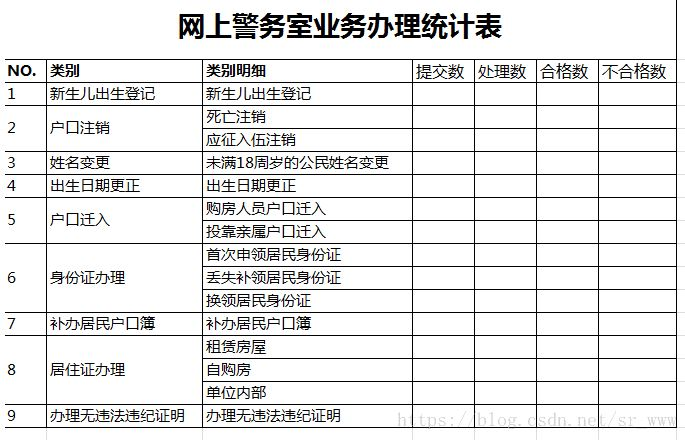select class1_id
,class1_name
,class2_id
,class2_name
,run_id
,count(run_id) as count_all#提交数
,count(run_ok>0 or null) as count_done#处理数
,count(run_ok=1 or null) as count1#合格数
,count(run_ok=2 or null) as count2#不合格数
from class2
join class1 on class2_class1_id=class1_id
left join run on run_class2_id=class2_id and date(run_addtime) between '$s_date1' and '$s_date2'
group by class2_id
order by class1_id,class2_id

输出结果：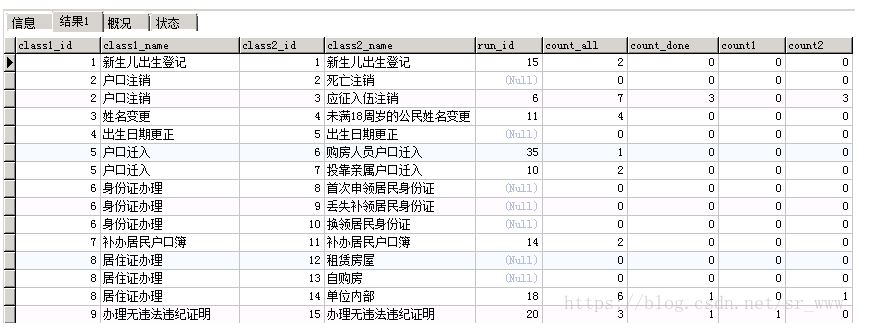展开全文mysql 数据库
• 某些情况下用count（*）来做计数是不能很好的实现的，这时候可以用sum配合case表达式来做累加计数，范例如下： SELECT   sum(case when rep_haddf = 1 then 1 else 0 end) ,  sum(case when rep_...
某些情况下用count（*）来做计数是不能很好的实现的，这个时候可以用sum配合case表达式来做累加计数，范例如下：
SELECT
sum(case when rep_haddf = 1 then 1 else 0 end) ,
sum(case when rep_progress = 100 then 1 else 0 end)
FROM dailly_report
where rep_type = '计划'
group by rep_userid
前一列计算出 条件符合： 是计划且rep_haddf = 1 的记录数
后一列计算出 条件符合： 是计划且rep_progress = 100 的记录数
所有数据按 rep_userid 为区分列出。
case表达式判断条件，如何条件的输出1，不符合的输出0，通过sum（）函数累加就实现了计数的功能，这种时候如果用count（*）就不好写了。

展开全文• 现在有一张student表，包含三字段：s_id，c_id，s_score。 目的1：只保留得到s_id为01，s_score为80的结果 输入语句： select * from score where case when s_id =01 and s_score =80 then 0 else 1 end= 0; ...
原始表
现在有一张student表，包含三个字段：s_id，c_id，s_score：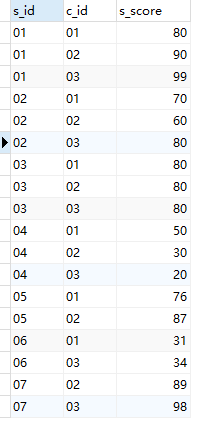目的1：如果只保留得到s_id为01，s_score为80的结果
输入语句：
select * from score where case when s_id =01 and s_score =80 then 0 else 1 end=
0;

结果：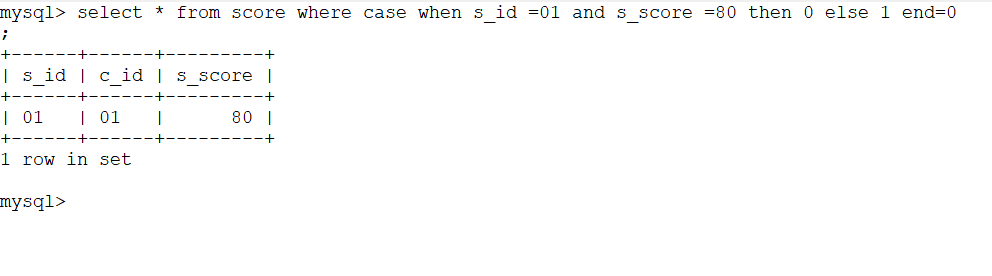目的2：筛选除同时满足s_id为01，s_score为80以外的所有数据
输入语句：
select * from score where case when s_id =01 and s_score =80 then 0 else 1 end=
1;

输出结果：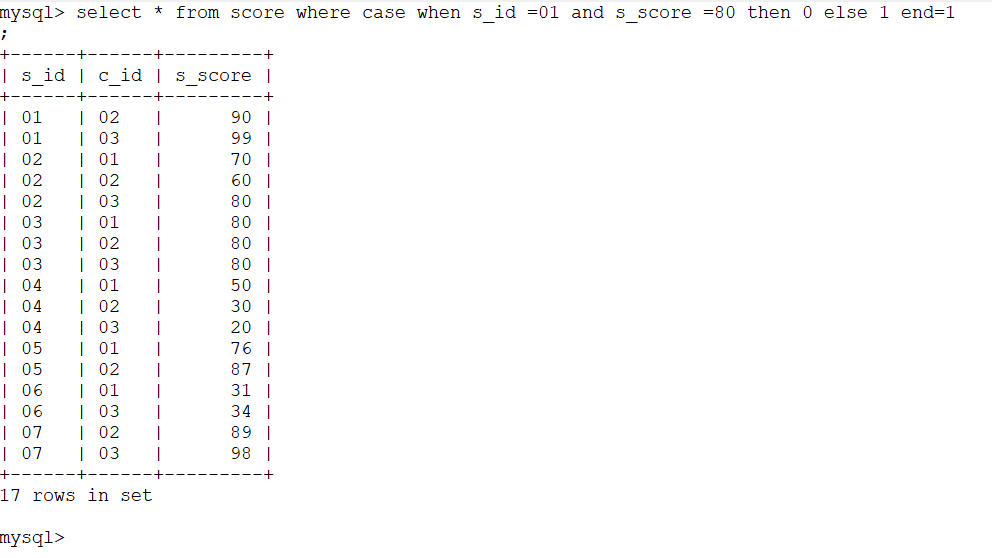展开全文SQL 过滤
• sum + case when / decode code select sum (decode (t.status, 'T', 1, 0)) t_status, sum(case when t.dat=sysdate then 1 else 0 end) now_date from (select 1 id, 'T' status, sysdate-1 dat from dual un....
• 前面段充分体现了博主深厚的文学素养，别和博主争，博主说啥就是啥。 其实，对于大量数据的处理，这几年微软Office做的不单单是2007的时候把Excel的行数从65536提高到了1048576。存得多了不是目的，目的是处理...
• applyInfo： SELECT  SUM(  IF (  a.certificate_level_id = 'ZSLX001',  1,  0  )  ) AS typeA,  SUM(  IF (  a.certific
• 内容提要：文章总结excel 多条件计数的三种方法，分别通过SUMPRODUCT、COUNTIFS函数、数据透视表来完成多条件计数。 　excel 多条件计数的使用频率是很高的。如果用函数来进行多条件计数，可以用SUMPRODUCT和...
• 从前有一皇帝，他有50妃子，这些妃子很没有天理的给他生了100,000儿子，于是，皇帝很苦恼，海量的儿子很难管理，而且，他想知道每妃子给他生了多少儿子，从而论功行赏，这很难办。于是，皇帝请了一...mysql MySQL
• ## 计数原理

千次阅读 2018-03-11 11:48:27
现实问题1. 分类加法计数原理场景：从甲地到乙地，可以乘火车、汽车、轮船。火车有 4 班、汽车 2 班、轮船 3 班，那么一天中乘坐这些...分类加法计数原理概念：如果一目标可以在 n 种不同情况下完成，第 k 种情...数学
• 基本计数原理： @加法原理：设集合s划分为两两互不相交的各个部分s1,s2,s3……sn，那么s的元素总数等于各个部分的总数之和。即|s|=|s1|+|s2|+……+|sn|.（如果允许子部分相交，则需要用容斥原理来解决）使用...
• mysql一条sql查询多个不同条件下的count值（count(if())的用法）！！！ 案例 查询数据库中不同日期内的不同状态下的count： sql语句： select date(create_time) as dateTime,count(IF(status!=2,true,null)) as ...java mysql count
• 经常会遇到根据不同条件统计总数的问题，一般有种写法：count和sum都可以 数据准备： 方法一 ：Count 代码： SELECT COUNT( CASE WHEN age > 20 AND age < 25 THEN 1 ELSE NULL END ) ...
• 语法：COUNTIFS(criteria_range1, criteria1, criteria_range2, criteria2...SUMIFS和AVERAGEIFS语法和用法类似，只不过第一参数要写需要求和/平均值的Range。 以SUMIFS语法为例：SUMIFS(sum_range, criteria_ran...Excel Office Formula
• ## 引用计数

千次阅读 2007-07-29 13:06:00
这个技巧有两个常用动机。第一个是简化跟踪堆中的对象的过程。一旦一个对象通过调用new被分配出来，最要紧的就是记录谁拥有这个对象，因为其所有者－－并且只有其所有者－－负责对这个对象调用delete。但是，所有权...string class delete 数据结构 struct
• select a.dfsname, count(b.dfsid) as instnum from tbl_dfslist a left join tbl_instance b on a.id = b.dfsid group by a.id; （其中tbl_dfslist.id = tbl_instance.dfsid）联合查询
• 仅提供一种思路，其中参数（阈值，中间具体膨胀腐蚀次数需要根据不同情况进行调整） 目的：分别将图中四种细胞的数量及每种细胞的面积求出来。 其中用到的方法有阈值处理，腐蚀（最终腐蚀，条件腐蚀），膨胀...matlab
• 十六、比较两个样本 原文：Comparing Two Samples 译者：飞龙 协议：CC BY-NC-SA 4.0 自豪地采用谷歌翻译 最近邻分类方法的动机是这样的，个体可能像最近的邻居。 从另一个角度来看，我们可以说一个...
• 一、题目描述给定两个已经排序好的数组，找到两者所有元素中第k大的元素二、解法分析解法一：参照归并排序 将两个有序数组变成一个有序数组：merge两个数组，然后求第k大的数，时间复杂度O(m+n)解法二：游标计数 ...
• 计数排序是一非基于比较的排序算法，该算法于1954年由 Harold H. Seward 提出。它的优势在于在对一定范围内的整数排序时，它的复杂度为Ο(n+k)（其中k是整数的范围），快于任何比较排序算法。当然这是一种牺牲...排序算法 桶排序 java排序
• 定时器计数说白了就是把内部时钟信号（fmaster）变成外部时钟信号(TIx、ETR)，计数需要有时钟/触发控制器和时基单元，定时器1拥有这两个，定时器2和3没有，所以2、3不能用于外部信号计数。 使用外部信号计数有两种...
• 用四种不同颜色给三棱柱六个顶点涂色,要求每个点涂一种颜色,且每条棱的两个端点涂不同颜色,则不同的涂色方法有多少种？ 当我看完题目后，顿时不知所措。于是我拿起草稿纸在一旁漫无目的地演算了一下，企图能找到...数学 算法 python
• ## 计数排序算法

千次阅读 2007-01-07 12:15:00
计数排序是一个非基于比较的线性时间排序算法。... 在这两个条件下，计数排序的复杂性为O(n)。计数排序算法的基本思想是对于给定的输入序列中的每一个元素x，确定该序列中值小于x的元素的个数。一旦算法 delete c
• 计数排序算法介绍 比较排序算法可以通过决策树模型证明，其下线是O(nlgn)。...但是计数排序有两个限制条件，那就是存在一个正整数K，使得数组里面的所有元素的key值都不大于N，且key值都是非负整数。 计数排序算法J排序算法 Java
• ## java科学计数法

千次阅读 2017-03-17 19:25:00
1 科学计数法的概念 1.1 有效数字 在一近似数中，从左边第一不是0的数字起，到精确到的位数止，这中间的所有数字都叫做这近似数的有效数字 例如： 890314000保留三位有效数字为8.90×10的8次方 ...java
• 排列计数（permutation） 题目描述     求有多少种长度为n的序列A，满足以下条件： 1) 1~n这n个数在序列中各出现了一次 ...2) 若第i个数A[i]的值为i，则称i是...接下来T行，每行两个整数n、m。   输出   ...
• 两个字符串相等的条件如下，字符串中的每个字母出现的次数都相等。例如：abbcd和acdbb是相等的，因为a都出现1次，b都出现2次，c都出现3次，d都出现4次。请先给出解题的思路，然后用你熟悉的语言进行具体的代码实现。......